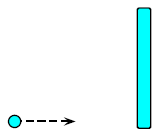# Problem: A sticky ball collides with and sticks to rod that is floating free in space. Consider the system of the ball and the rod, and let E be the kinetic energy of the system, p the linear momentum of the system, and L the angular momentum of the system. List which of these quantities are conserved about the point where the putty strikes the rod.1. p; E2. L3. L; E4. L; p; E5. E6. p7. L; p8. none

87% (23 ratings)
###### Problem Details

A sticky ball collides with and sticks to rod that is floating free in space. Consider the system of the ball and the rod, and let E be the kinetic energy of the system, p the linear momentum of the system, and L the angular momentum of the system. List which of these quantities are conserved about the point where the putty strikes the rod.

1. p; E

2. L

3. L; E

4. Lp; E

5. E

6. p

7. Lp

8. none